# Jig:Saw’s puzzle solutions

## Puzzle 1

Milton took the IQ, EQ and creativity tests that Zach took. His scores were IQ = 170, EI = 70 and creativity = 55. Calculate the z-scores relative to the population values in Table 6.1 (in the book). How do Milton’s scores compare to Zach’s?

Table 1: Zach’s and Milton’s raw scores on three tests converted to z-scores using the population means and standard deviations for each test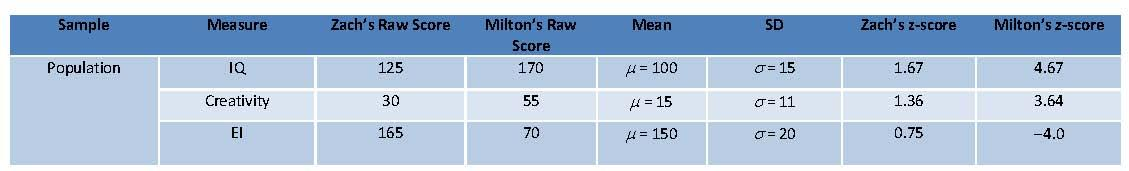For IQ Milton’s z-score would be: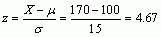For Creativity Milton’s z-score would be: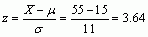For EI Milton’s z-score would be: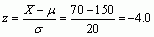The question also asks us to compare Zach and Milton’s z-scores. For IQ Zach got a z-score of 1.67, which is higher than the mean of zero indicating that Zach is more intelligent than the average person. However, Milton’s z-score of 4.67 is incredibly high, it is very unusual to see values outside of ±3 and extremely rare to see values outside of ±4; so, Milton is a genius! For creativity Zach got a similar z-score to the one he got for IQ, he scored z = 1.36, which again puts him above average for this trait, but once again Milton has beaten Zach by quite a large margin by scoring z = 3.64, which again is a very high score and also implies that Milton is a genius. However, the story is quite different when comparing Zach and Milton on emotional intelligence; for this trait Zach got a z-score of 0.75, which puts him a bit above average, whereas Milton scored terribly, he got a z –score of -4, which is about as far below the mean as you can get, suggesting he has basically no emotional intelligence at all!

## Puzzle 2

In the file in Dr Genari’s office, Zach also found that Alice had a score of 57 out of 60 on an IQ test (a different one than the one Zach completed). This test had a mean of 32 and a standard deviation of 7. Using this information and the answer to the previous puzzle, who has a larger IQ: Alice or Milton?

First we need to convert Alice’s IQ score of 57 into a z-score by using the equation below:Therefore, for IQ Alice scored z = 3.57 and looking at the previous question we can see that Milton scored z = 4.67. Both z-scores are above 3, suggesting that Alice and Milton are both a lot more intelligent than the average person but Milton has the higher IQ.

## Puzzle 3

When Zach met Professor Pincus (Chapter 3), she showed him data from 20 women who rated how important they found various characteristics in men. They rated from 0 (not at all important) to 10 (very important) characteristics such as high salary, humour, kindness and ambition. The data are in Table 3.1 (in the book). A student outside Occam’s asked Celia to rate how important these same characteristics were in a partner. She gave high salary a rating of 5. What is this value as a z-score? (Hint: use the values in Table 3.1 to compute the mean and standard deviation of the distribution of high salary ratings.)

First we need to compute the mean: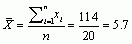Then the standard deviation as we have done before (the scores for high salary are from Table 3.1 in the book):

Table 2: Calculating the sum of squares for high salaryTo calculate the variance, use the sum of squares from Table 2: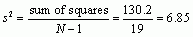We can now calculate the standard deviation:Now we can convert Celia’s rating of 5 for the high salary variable into a z-score by plugging the mean, standard deviation and Celia’s score of 5 into the equation for calculating z-scores: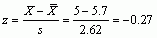Therefore, Celia’s z-score for high salary is –0.27, which is a little bit below the mean. This suggests that Celia doesn’t value high salary as a particularly important characteristic in a romantic partner.

## Puzzle 4

For kindness, Celia’s rating yielded a z-score of 0.9. What was her raw score? (Hint: use the values in Table 3.1 in the book to compute the mean and standard deviation of the distribution of kindness ratings.)

First, we compute the mean:Then compute the standard deviation as we have done before (the scores for kindness are from Table 3.1).

Table 3: Calculating the sum of squares for kindness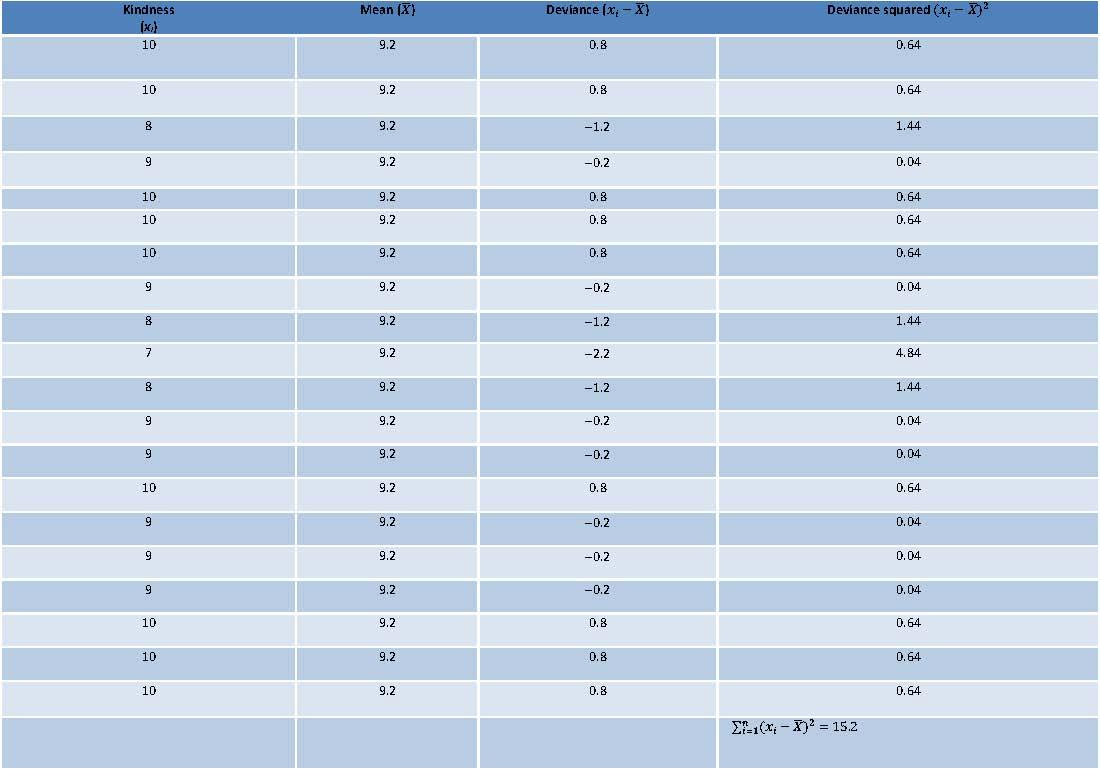To calculate the variance use the sum of squares from Table 3: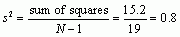We can now calculate the standard deviation:Now we can convert Celia’s z-score for the kindness variable back into her raw score by rearranging the equation for z-scores, and then plugging in her z-score and the values of the mean and standard deviation that we have just calculated: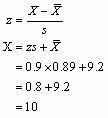Therefore, Celia’s raw score for the kindness was 10 out of 10, suggesting that Celia values kindness very highly in a romantic partner.

## Puzzle 5

For ambition, Celia gave a rating of 9, which yielded a z-score of 1.56. What was the standard deviation of ambition ratings? (Hint: use the values in Table 3.1 in the book to compute the mean of the distribution of ambition ratings.)

First we need to compute the mean:We can calculate the standard deviation (s) of the ambition ratings by rearranging the equation for finding the z-score and then plugging in the values of z, Celia’s rating (X) and the mean (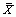) that are provided in the question: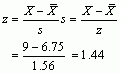Therefore, the standard deviation for ambition was 1.44.

## Puzzle 6

For humour, Celia also gave a rating of 9, which yielded a z-score of 0.66. The standard deviation of humour scores was 1.43. What was the mean of the humour ratings?

We can calculate the mean () of the humour ratings by rearranging the equation for finding z-scores and then plugging in the values of z, Celia’s rating of humour (X) and the standard deviation (s) that are provided in the question: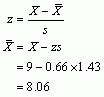Therefore, the mean rating for humour was 8.06 out of 10.

## Puzzle 7

Which of the four attributes did Celia value most? (Hint: use the z-scores.)

I have put Celia’s z-scores for each characteristic into a table so that we can compare them most easily (Table 4). If we look at Celia’s raw scores we can see that she gave the highest rating for kindness (10 out of 10) and the lowest rating for high salary (5 out of 10). However, if we look at her z-scores we can see her ratings of the four characteristics in relation to the ratings of the 20 other women. When we do this we can see that her highest z-score by far was for ambition, which was 1.56, suggesting that compared to the other women in the study, Celia values ambition very highly. Her lowest z-score was for high salary (-0.27); this negative z-score suggests that compared to the other women in the study, Celia does not value high salary as a very important characteristic in a partner.

Table 4: Celia’s raw scores and z-scores for ratings of four characteristics she might value in a mate## Puzzle 8

Celia mentioned Zach’s cheekbones a lot. In the data Professor Pincus looked at there were also 20 women’s ratings of how important they thought attractiveness was. The scores were: 4, 10, 9, 8, 7, 8, 10, 8, 7, 3, 9, 10, 8, 10, 7, 9, 9, 9, 8, 7. Convert this distribution to z-scores. Celia rated attractiveness as 9. What was the z-score for this raw score?

Table 5: Calculating the sum of squares for attractiveness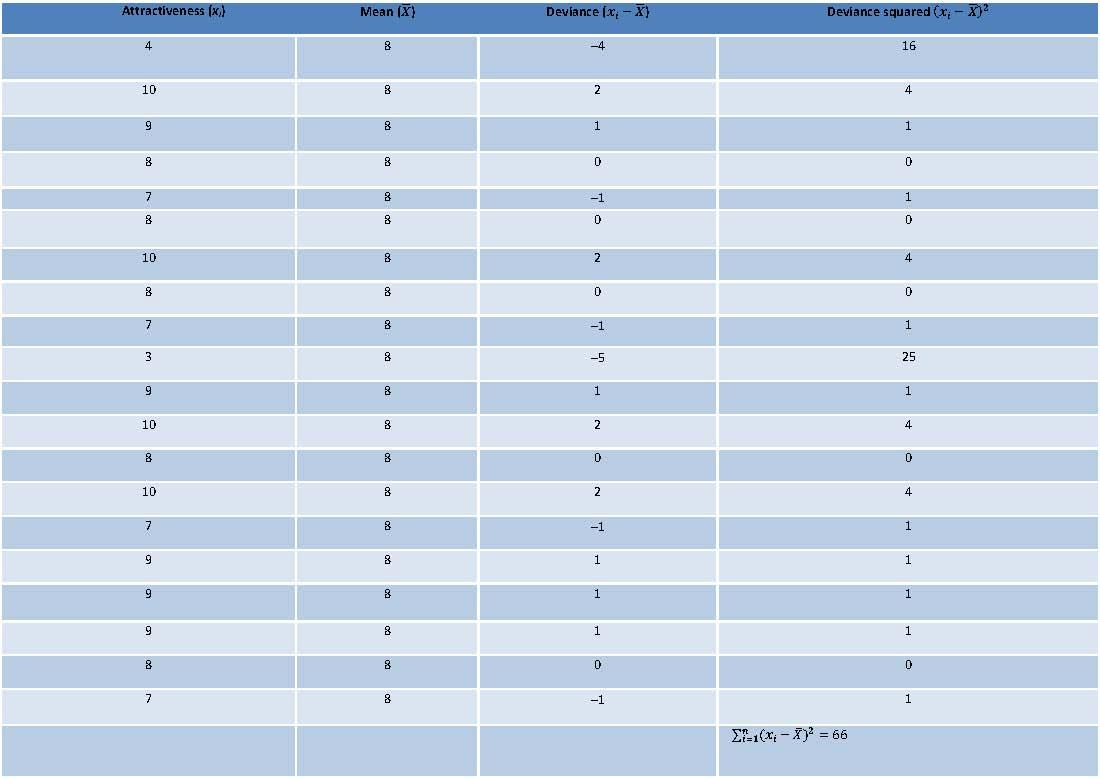To calculate the variance use the sum of squares in Table 5: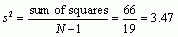We can now calculate the standard deviation: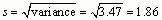Now we know what the mean and standard deviation of the distribution are, we can work out the z-scores of each of the raw scores by using the equation: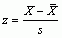The scores were 3,4,7,8,9, and 10: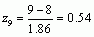The distribution, therefore, becomes as shown in Table 6.

Table 6: Distribution for the variable ‘attractiveness’ converted to z-scores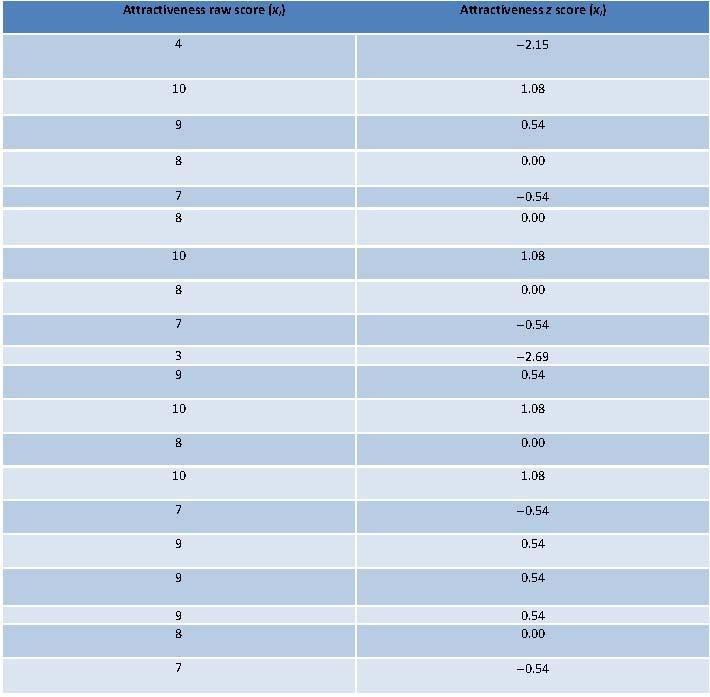The z-score for Celia’s raw score of 9 was 0.54. This positive z-score suggests that Celia rates attractiveness as quite an important characteristic in a partner.

## Puzzle 9

Alice also rated these characteristics of a partner, but using a different rating scale. For kindness, this scale had a mean of 15 and a standard deviation of 7. Alice rated kindness as 21. Who values kindness more: Alice or Celia? (Hint: compare the z-scores.)

First we need to convert Alice’s raw score into a z-score:Therefore, Alice’s z-score for kindness was 0.86, and if we look back at Celia’s z-score for kindness we can see that it was 0.9. These scores are almost identical, indicating that Alice and Celia value kindness very similarly.

## Puzzle 10

In the data Professor Pincus looked at there were also 20 women’s ratings of how important they thought being romantic was. The scores were: 7, 10, 7, 8, 9, 8, 10, 7, 7, 4, 5, 8, 7, 8, 7, 9, 3, 7, 10, 7. Convert this distribution to z-scores. Celia rated being romantic a rating of 9. what was the z-score for this raw score?

Table 7: Calculating the sum of squares for ‘Romantic’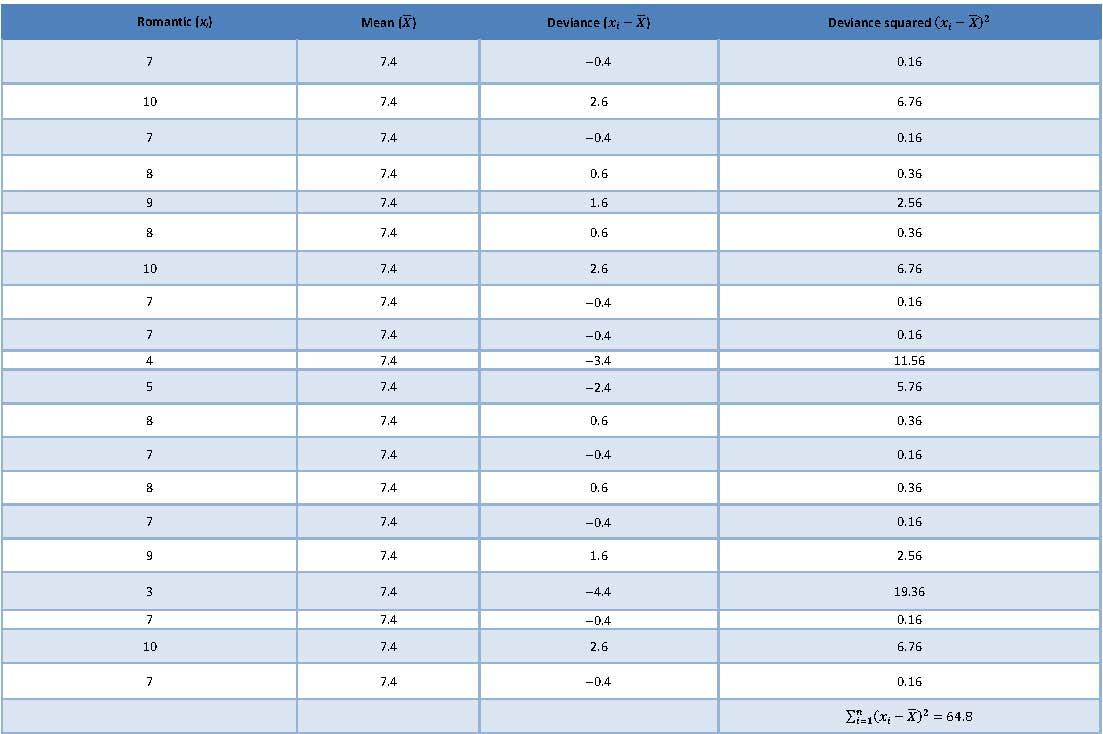To calculate the variance, use the sum of squares from Table 7: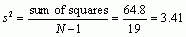We can now calculate the standard deviation: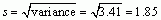Now we know what the mean and standard deviation of the distribution are, we can work out the z-scores of each of the raw scores by using the equation:The distribution, therefore, becomes as shown in Table 8.

Table 8: Distribution for the variable ‘romantic’ converted to z-scores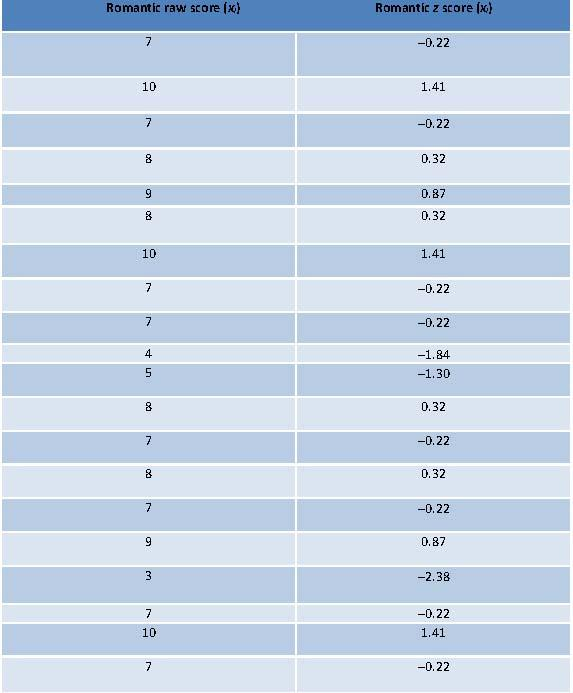The z-score of Celia’s raw score of 9 was 0.87, suggesting that she values the characteristic romantic  in a partner more than the average person (nearly a standard deviation higher, in fact).